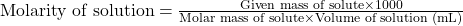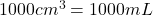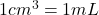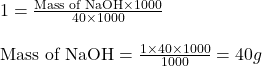## how many grams of NaOH will make 1M of its aqueous solution in 1000cm3

Question

how many grams of NaOH will make 1M of its aqueous solution in 1000cm3

in progress 0
6 months 2021-07-22T02:07:46+00:00 1 Answers 6 views 1

## Answers ( )

1. Answer: The mass of NaOH is 40 g

Explanation:

Molarity is defined as the amount of solute expressed in the number of moles present per liter of solution. The units of molarity are mol/L. The formula used to calculate molarity:…..(1)

Given values:

Molarity of NaOH = 1 M

Molar mass of NaOH = 40 g/mol

Volume of the solution =(Conversion factor:)

Putting values in equation 1, we get:Hence, the mass of NaOH is 40 g